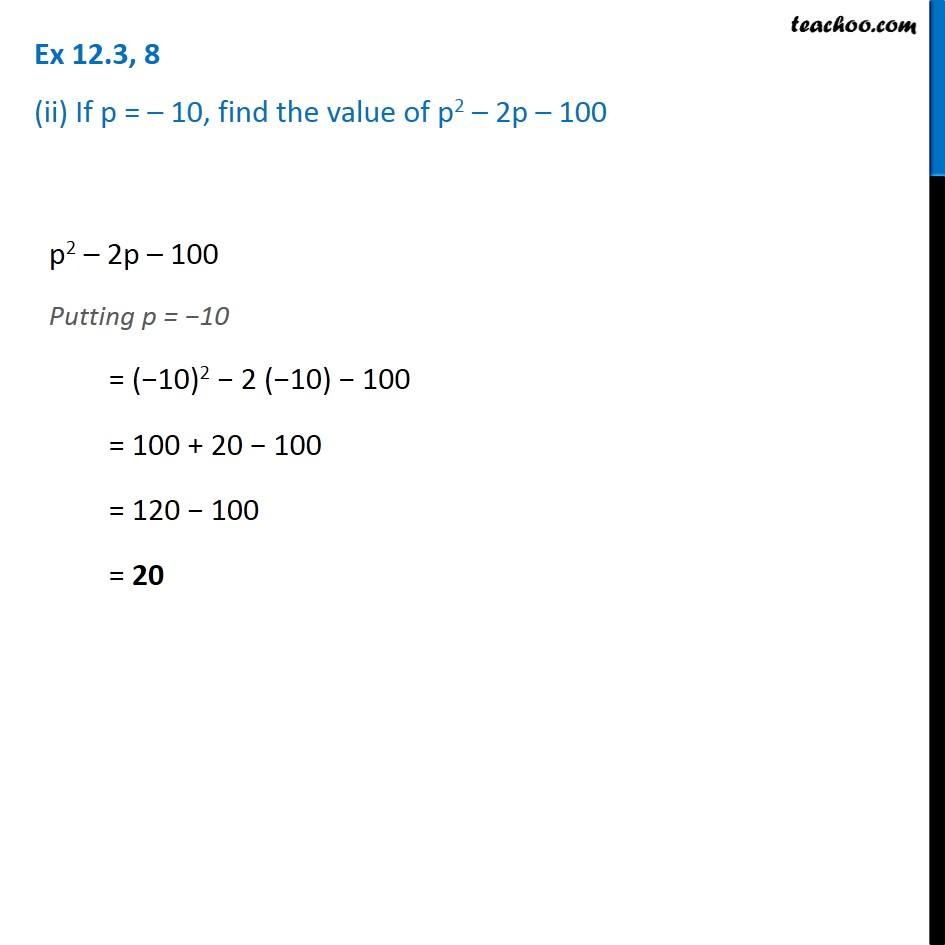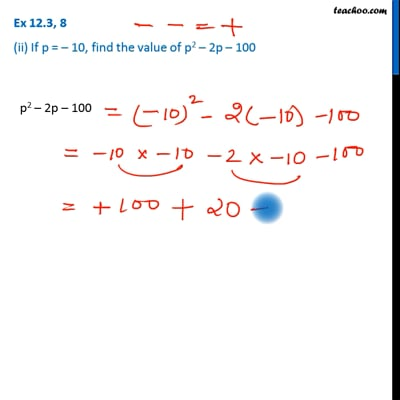Ex 12.3

Chapter 12 Class 7 Algebraic Expressions
Serial order wiseThis video is only available for Teachoo black users

Get live Maths 1-on-1 Classs - Class 6 to 12

### Transcript

Ex 12.3, 8 (ii) If p = – 10, find the value of p2 – 2p – 100 p2 – 2p – 100 Putting p = −10 = (−10)2 − 2 (−10) − 100 = 100 + 20 − 100 = 120 − 100 = 20